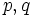# Order has only two prime factors implies solvable for Moufang loops

Suppose$L$ is a finite Moufang loop (i.e., a Moufang loop whose underlying set is finite). Suppose the order (i.e., the size of the underlying set) of$L$ has at most two distinct prime factors, i.e., it is of the form$p^aq^b$ where$p,q$ are primes and$a,b$ are nonnegative integers (possibly zero). Then,$L$ is a Solvable Moufang loop (?) (and in particular a finite solvable Moufang loop).